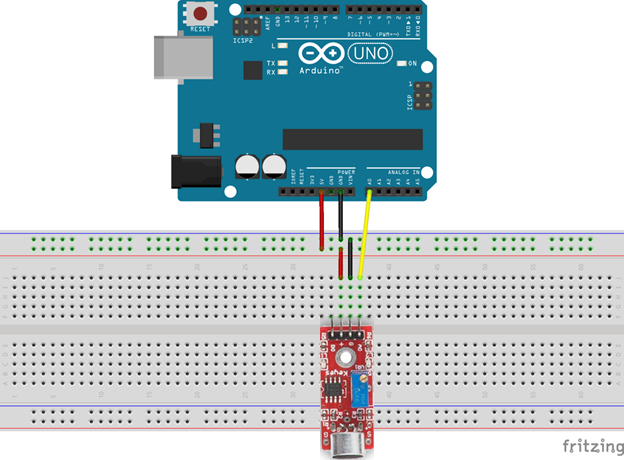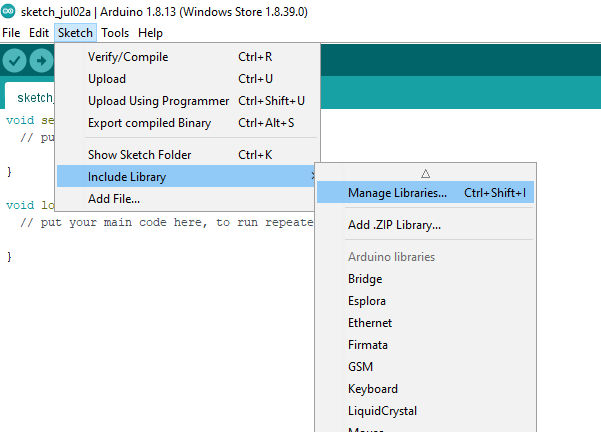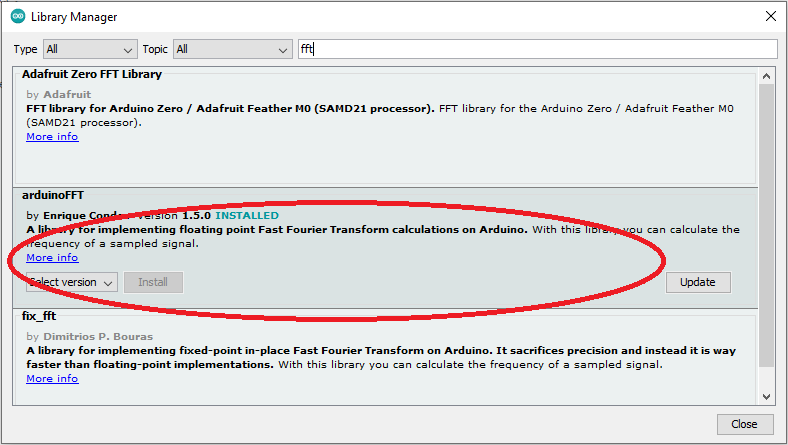# My Weekend Project: Audio Frequency Detector Using An Arduino

Description: This Arduino project displays the approximate frequency of the loudest sound detected by a sound detection module. For this project, the analog output from the sound module detector sends the analog audio signal detected to A0 of the Arduino Uno. The analog signal is sampled and quantized (digitized). A Fast Fourier Transform (FFT) is then performed on the digitized data. The FFT converts the digital data from the approximate discrete-time domain result. The maximum frequency of the approximate discrete-time domain result is then determined and displayed via the Arduino IDE Serial Monitor.

Parts List:

Fritzing Diagram: Below is a Fritzing Diagram of the circuit constructed for this project.Figure 1

Obtain the arduinoFFT Library: To obtain the arduinoFFT library, open your Arduino IDE.

1. Go to Sketch>Include Library>Manage Libraries…(See Figure 2)
2. Type fft in the search box and install arduinoFFT (See Figure 3)Figure 2Figure 3

Obtain the Code (aka Sketch): You may get the code by going to this page, Arduino Frequency Detector Code. Do not worry, it is free.